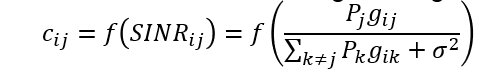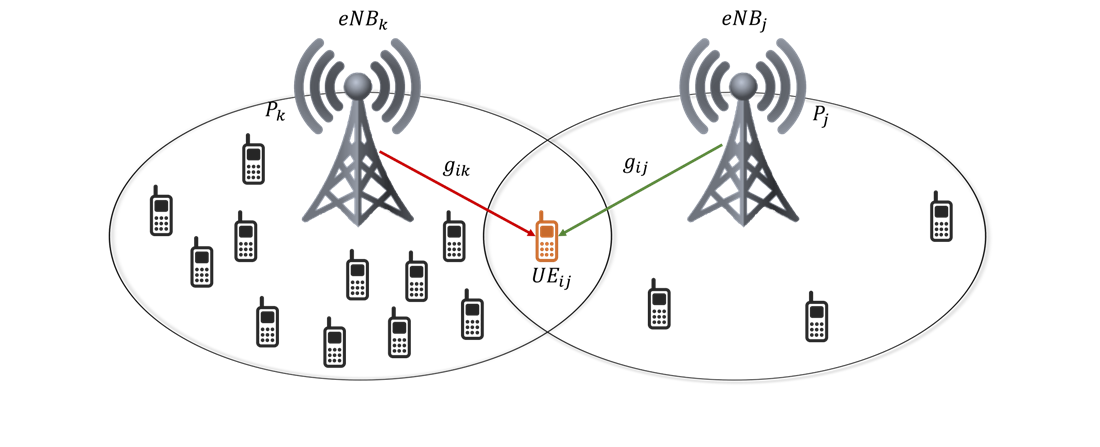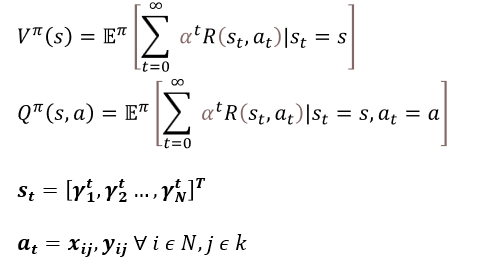Given below is a high-level description of an example of how RL can be used with NetSim

Mobility load balancing in LTE/5G

• Load transfer from an overloaded cell to an under-loaded neighbouring cell• c_ij is the instantaneous rate of a UE and is theoretically a log function of SINR• R_ij is the long-term rate and y_ij is the fraction of resources allocated, to UE_i by BS_j• RL will be required if SINR changes with time
• User mobility / Random network topology
• DL transmit power variation
• Markov decision process/Q-learning based (model-free) RL
• At state s_t  RL agent selects action a_t by following policy π and receives reward r(s_t, a_t).
• The MDP has value function V^π (s), and action value function Q^π (s, a) where α (0≤α≤1) is the discount factor
• Update interval (epoch) ≫ LTE frame length
• State: UE SINRs (γ_1,…, γ_N  ), based on the current association at time t
• Action: Association x_ij, Resource allocation y_ijVariations

• Consider GBR and Non GBR users
• Split between GBR PRB usage and Non GBR PRB usage
• Time-varying network traffic
• Use deep neural networks to approximate the Q and Value functions
• Additional constraint: Minimum throughput per user (i.e., minimum SINR , γ, to all users)
• Objective: Latency minimization

Other examples

• Association based on logical cell boundaries using Cell individual offset (CIO)
• Power control in multi-tier HetNets